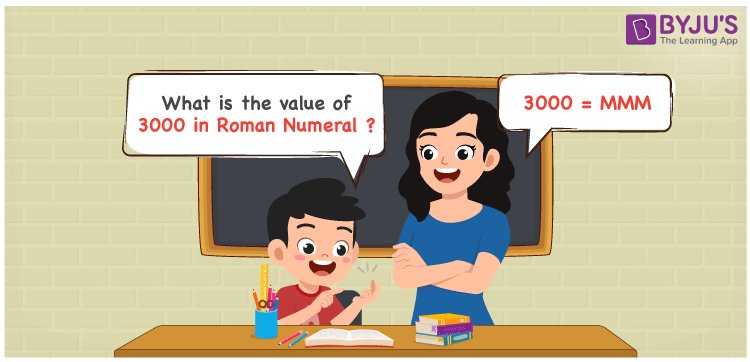# 3000 in Roman Numerals

MMM is 3000 in Roman numerals. To convert 3000 to Roman Numerals, first write 3000 in expanded form, i.e. 3000 = 1000 + 1000 + 1000, then replace the changed numbers with corresponding roman numerals to get 3000 = M + M + M = MMM. In this article, we’ll explain to you how to convert 3000 to Roman numerals correctly.

 Number Roman Numeral 3000 MMM

## How to Write 3000 in Roman Numerals?In order to write 3000 in Roman Numerals, it has to be written in expanded form, i.e.

3000 = 1000 + 1000 + 1000

3000 = M + M + M = MMM.

3000 = MMM

## Video Lesson on Roman Numerals